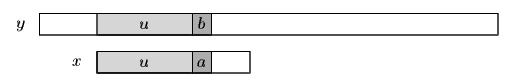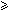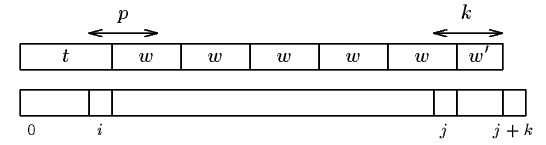Next: Optimal Mismatch algorithm Up: ESMAJ Prev: Two Way algorithm

# String Matching on Ordered Alphabets

Main features
• no preprocessing phase;
• requires an ordered alphabet;
• constant extra space complexity;
• searching phase in O(n) time;
• performs 6n+5 text character comparisons in the worst case.
Description

During an attempt where the window is positioned on the text factor y[j .. j+m-1], when a prefix u of x has been matched and a mismatch occurs between characters a in x and b in y (see figure 26.1), the algorithm tries to compute the period of ub, if it does not succeed in finding the exact period it computes an approximation of it.Figure 26.1: Typical attempt during the String Matching on an Ordered Alphabet algorithm.

Definition
Let us define twew' the Maximal-Suffix decomposition (MS-decompostion for short) of the word x if:v = wew' is the maximal suffix of x according to the alphabetical ordering;w is basic;e1;w' is a proper prefix of w.

Then we have |t| < per/>(x).

If twew' is the MS-decomposition of a nonempty word x then the four properties hold:if t is a suffix of w then per(x) = per(v);per(x) > |t|;if |t||w| then per(x) > |v| = |x|-|t|;if t is not a suffix of w and |t| < |w| then per(x) > min(|v,|twe|).

If u is a suffix of w then per(x)=per(v)=|w|.
Otherwise per(x) > max(|u,min(|v|, |twe|))|x|/2.

If twew' is the MS-decomposition of a nonempty word x, per(x) = |w| and e > 1 then If twe-1w' is the MS-decomposition of x' = uwe-1w'.

The algorithm computes the maximal suffix of the matched prefix of the pattern appended with the mismatched character of the text after each attempt. It avoids to compute it from scratch after a shift of length per(w)\$ has been performed.

The String Matching on Ordered Alphabets needs no preprocessing phase.

The searching phase can be done in O(n) time complexity using a constant extra space. The algorithm performs no more than 6n+5 text character comparisons.

The C codeFigure 26.2: Meaning of the variables i, j, k, p in the function NEXT_MAXIMAL_SUFFIX.

```/* Compute the next maximal suffix. */
void nextMaximalSuffix(char *x, int m,
int *i, int *j, int *k, int *p) {
char a, b;

while (*j + *k < m) {
a = x[*i + *k];
b = x[*j + *k];
if (a == b)
if (*k == *p) {
(*j) += *p;
*k = 1;
}
else
++(*k);
else
if (a > b) {
(*j) += *k;
*k = 1;
*p = *j - *i;
}
else {
*i = *j;
++(*j);
*k = *p = 1;
}
}
}

/* String matching on ordered alphabets algorithm. */
void SMOA(char *x, int m, char *y, int n) {
int i, ip, j, jp, k, p;

/* Searching */
ip = -1;
i = j = jp = 0;
k = p = 1;
while (j <= n - m) {
while (i + j < n && i < m && x[i] == y[i + j])
++i;
if (i == 0) {
++j;
ip = -1;
jp = 0;
k = p = 1;
}
else {
if (i >= m)
OUTPUT(j);
nextMaximalSuffix(y + j, i+1, &ip, &jp, &k, &p);
if (ip < 0 ||
(ip < p &&
memcmp(y + j, y + j + p, ip + 1) == 0)) {
j += p;
i -= p;
if (i < 0)
i = 0;
if (jp - ip > p)
jp -= p;
else {
ip = -1;
jp = 0;
k = p = 1;
}
}
else {
j += (MAX(ip + 1,
MIN(i - ip - 1, jp + 1)) + 1);
i = jp = 0;
ip = -1;
k = p = 1;
}
}
}
}

```
The example

Searching phase

References
• CROCHEMORE M., 1992, String-matching on ordered alphabets, Theoretical Computer Science 92(1):33-47.
• CROCHEMORE, M., RYTTER, W., 1994, Text Algorithms, Oxford University Press.Next: Optimal Mismatch algorithm Up: ESMAJ Prev: Two Way algorithm

e-mails: {Christian.Charras, Thierry.Lecroq }@laposte.net
Tue Jan 14 15:03:31 MET 1997